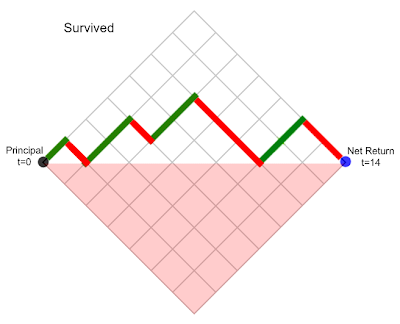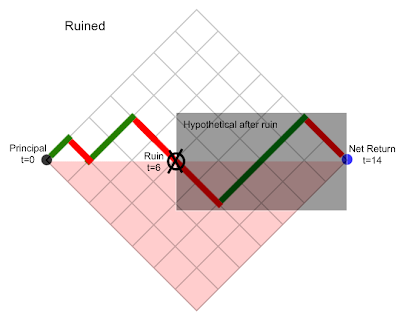# Probability of RuinMarch 1, 2009
634 Views

Catalan Numbers are an interesting way of modeling the probability of ruin.

Specifically, Catalan Numbers help find the probability that a Brownian process such as equity prices will have more losers than winners at any time before the end of the period being tested. So if three trades are good and then four go bad, we would call this “ruin” because we are below the baseline. Now, for example, let’s say we want to know the odds of ruin over any 14 trades with a winning percentage of 50%. So the outcomes may look as follows (time is the horizontal and return vertical):The formula for the probability of survival is as follows, where n=14/2=7 in this caseSolving, P=439/5040=8.5%. So with a 50/50 chance of a winning trade, you will almost certainly be down at some point during a 14 trade period.

Some of the assumptions that need to be eliminated are: the exact balancing of winning and losing trades (but in different orders), 50% odds, and the definition of ruin being down by a net of just one bet (or, equivalently, having higher starting principal than 1 wager). I will keep researching what has been done to compesate for these assumptions. The theory I’ve described so far has apparently

Catalan Numbers are an interesting way of modeling the probability of ruin.

Specifically, Catalan Numbers help find the probability that a Brownian process such as equity prices will have more losers than winners at any time before the end of the period being tested. So if three trades are good and then four go bad, we would call this “ruin” because we are below the baseline. Now, for example, let’s say we want to know the odds of ruin over any 14 trades with a winning percentage of 50%. So the outcomes may look as follows (time is the horizontal and return vertical):The formula for the probability of survival is as follows, where n=14/2=7 in this caseSolving, P=439/5040=8.5%. So with a 50/50 chance of a winning trade, you will almost certainly be down at some point during a 14 trade period.

Some of the assumptions that need to be eliminated are: the exact balancing of winning and losing trades (but in different orders), 50% odds, and the definition of ruin being down by a net of just one bet (or, equivalently, having higher starting principal than 1 wager). I will keep researching what has been done to compesate for these assumptions. The theory I’ve described so far has apparently been known since 1844 so there are probably extensions floating around. One document I found already, via MIT OCW (an amazing resource; in high school I watched almost the entire Linear Algebra lecture series– good times), introduces what resembles the probability density function of a binomial random variable. This may eliminate the 50/50 odds assumption but I don’t completely understand it yet.

I think this is probably a better way to quantify risk than with VaR (i.e. standard deviation of periodic returns). In the Ralph Vince book I mentioned previously it lays out another model of a trader’s risk in terms of drawdown. It was also interesting but possibly limited by over-reliance on historical maximum drawdown. Of course every model is hamstrung by being forced to always look backwards like fortune tellers in The Inferno.

I’d like to know of any extensions of Catalan Numbers specifically to modeling risk in betting processes. As always, feel free to leave a comment on anything else too.